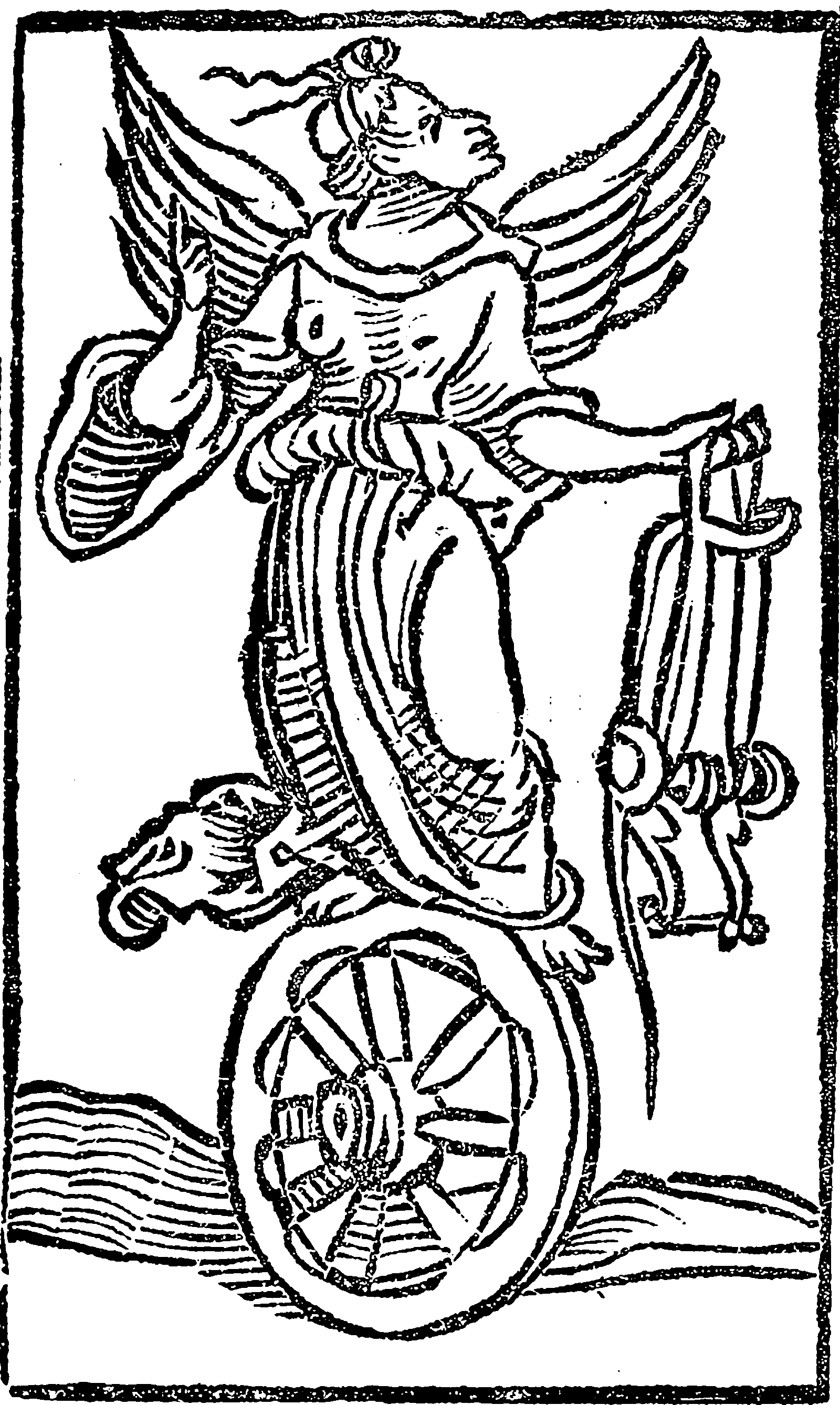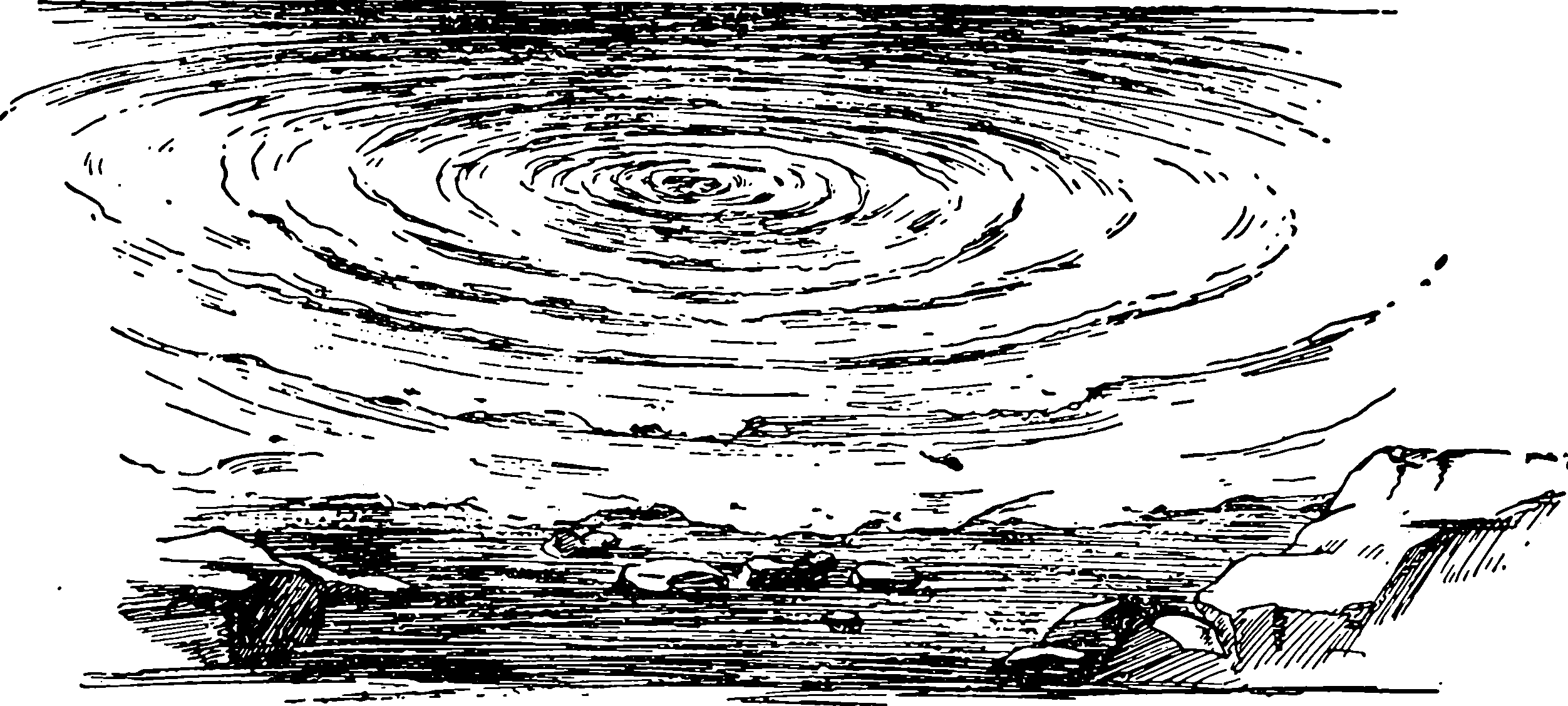A function $$g: \mathbb{R}^{d}/ {0} \rightarrow \mathbb{R}$$ is radial if there is a function $$k : \mathbb{R}^+ \rightarrow \mathbb{R}$$ such that $g(x)=k(\|x\|),\,x\in\mathbb{R}^d/\{0\}.$ We need to handle these things a lot, in arbitrary dimensions.

Let us put another way. Consider the $$n$$-dimensional polar coordinates representation of a vector, which is unique for non-null vectors: $x=r x^{\prime}, \quad \text { where } \quad r=\|x\| \quad \text { and } \quad x'=\frac{x}{\|x\|}.$ Radial functions are those which do not depend upon “angle” vector $$x'$$. These are important in spherical distributions.

Throughout this we will use spheres and balls a lot. Le us name them: We call the $$n$$-sphere, $$S_{n}(r):=\{x:\|x\|=r\}$$ and the $$n$$-ball $$B_{n}(r):=\{x:\|x\|\leq r\}.$$ note that $$x'\in S_{n}(1)$$.

## Basic spherical integrals

is a brutally minimalist introduction to integrating functions over a sphere, shorn of all fluff. He explains: Let $$\sigma$$ denote the $$(n-1)$$ -dimensional surface measure on $$S_{n}.$$ The formula for integration in polar coordinates is then $\int_{\mathbb{R}^{n}} f(x) d x=\int_{S_{n}} \int_{0}^{\infty} f\left(r x^{\prime}\right) r^{n-1} d r d \sigma\left(x^{\prime}\right)$ Our object is to compute $$\int_{S_{n}} P(x) d \sigma$$ where $$P$$ is a polynomial in the elements of $$x=[x_1\, x_2\, \dots \, x_n]^\top$$. For this it suffices to consider the case where $$P$$ is a monomial, $P(x)=x^{\alpha}=x_{1}^{\alpha_{1}} x_{2}^{\alpha_{2}} \cdots x_{n}^{\alpha_{n}} \quad\left(\alpha_{1}, \ldots, \alpha_{n} \in\{0,1,2, \ldots\}\right)$

Let $$\beta_{j}=\frac{1}{2}\left(\alpha_{j}+1\right) .$$ Then, he shows, $\int_{S_{n}} P d \sigma=\left\{\begin{array}{ll} 0 & \text { if some } \alpha_{j} \text { is odd, } \\ \frac{2 \Gamma\left(\beta_{1}\right) \Gamma\left(\beta_{2}\right) \cdots \Gamma\left(\beta_{n}\right)}{\Gamma\left(\beta_{1}+\beta_{2}+\cdots+\beta_{n}\right)} & \text { if all } \alpha_{j} \text { are even. } \end{array}\right.$

## As dot-product kernels

Radial functions are connected to dot product kernels, in that dot product kernels are a special case of radial functions. Working out whether a given radial function is also a dot-product kernel, i.e. that it is positive definite is not trivial. find rules for functions constrained to a sphere based on a Legendre basis decomposition. Alternatively you can use the constructive approach of the Schaback-Wu transform algebra which promises to preserve positive-definite-ness under certain operations. Both these approaches get quite onerous except in special cases.

## Transforms

How to work with radial functions.### Hankel transforms

A classic transform for dealing with general radial functions: $(\mathcal{H}_{\nu }k)(s):=\int _{0}^{\infty }k(r)J_{\nu }(sr)\,r\,\mathrm{d} r.$ Nearly simple. Easy in special cases. Otherwise horrible. TBC.

### Transform algebra

So here is a weird rabbit hole I went down; it concerns a cute algebra over radial function integrals that turns out to be not that useful for the kinds of problems you face, but comes out very nicely if you, e.g. have very particular function structures, or really want to know that you have preserved positive-definiteness in your functions.

Here I am going to try to understand , who handle the multivariate Fourier transforms and convolutions of radial functions through univariate integrals, which are a kind of warped Hankel transform. This is a good trick if it works, because this special case is relevant to, e.g. isotropic stationary kernels. They tweak the definition of the radial functions. Specifically, they call function $$g: \mathbb{R}^{d}/ {0} \rightarrow \mathbb{R}$$ is radial if there is a function $$f: \mathbb{R}^+ \rightarrow \mathbb{R}$$ such that $g(x)=f(\|x\|_2^2/2),\,x\in\mathbb{R}^d/\{0\}.$ This relates to the classic version by $$k(\sqrt{2s})=f(s).$$

is one of those articles where the notation is occasionally amiguous clear and it would have been useful to mark which variables are vectors and which scalars, and overloading of definitions. Also they recycle function names: watch out for $$f,$$ $$g$$ and $$I$$ doing double duty. They use the following convention for a Fourier transform: $\mathcal{F}_{d}g(\omega) := \hat{g}(\omega):=(2 \pi)^{-d / 2} \int_{\mathbb{R}^{d}} g(x) \mathrm{e}^{-\mathrm{i} \omega^{\top} x} \mathrm{~d} x$ and $\mathcal{F}^{-1}_{d}\check{g}(x):=(2 \pi)^{-d / 2} \int_{\mathbb{R}^{d}} g(\omega) \mathrm{e}^{+\mathrm{i} \omega^{\top} x} \mathrm{~d}(t)$ for $$g \in L_{1}\left(\mathbb{R}^{d}\right).$$

Now if $$g(x)=f\left(\frac{1}{2}\|x\|^{2}\right)$$ is a radial function, then the $$d$$-variate Fourier transform is \begin{aligned} \hat{g}(\omega) &=\|\omega\|_{2}^{-(d-2)/2} \int_{0}^{\infty} f\left(\frac{1}{2} s^{2}\right) s^{d / 2} J_{(d-2)/2}\left(s \cdot\|\omega\|_{2}\right) \mathrm{d} s \\ &=\int_{0}^{\infty} f\left(\frac{1}{2} s^{2}\right)\left(\frac{1}{2} s^{2}\right)^{(d-2)/ 2}\left(\frac{1}{2} s \cdot\|\omega\|_{2}\right)^{(d-2) / 2} J_{(d-2) / 2}\left(s \cdot\|\omega\|_{2}\right) s \mathrm{~d} s \\ &=\int_{0}^{\infty} f\left(\frac{1}{2} s^{2}\right)\left(\frac{1}{2} s^{2}\right)^{(d-2) / 2} H_{(d-2)/ 2}\left(\frac{1}{2} s^{2} \cdot \frac{1}{2}\|\omega\|_{2}^{2}\right) s \mathrm{~d} s \end{aligned} with the functions $$J_{\nu}$$ and $$H_{r}$$ defined by $\left(\frac{1}{2} z\right)^{-\nu} J_{\nu}(z)=H_{\nu}\left(\frac{1}{4} z^{2}\right)=\sum_{k=0}^{\infty} \frac{\left(-z^{2} / 4\right)^{k}}{k ! \Gamma(k+\nu+1)}=\frac{F_{1}\left(\nu+1 ;-z^{2} / 4\right)}{\Gamma(\nu+1)}$ for $$\nu>-1$$. (What on earth do they mean by the two argument form $$F_1(\cdot; \cdot)?$$ Is that a 1st-order Hankel transform?) If we substitute $$t=\frac{1}{2} s^{2},$$ we find \begin{aligned} \hat{g}(\omega)&=\int_{0}^{\infty} f(t) t^{(d-2) / 2} H_{(d-2)/2}\left(t \cdot \frac{1}{2}\|\omega\|^{2}\right) \mathrm{d} t \\ &=:\left(F_{\frac{d-2}{2}} f\right)\left(\|\omega\|^{2} / 2\right) \end{aligned} with the general operator \begin{aligned} \left(F_{\nu} f\right)(r) &:=\int_{0}^{\infty} f(t) t^{\nu} H_{\nu}(t r) \mathrm{d} t. \end{aligned}

$$F_{\frac{d-2}{2}}$$ is an operator giving the 1-dimensional representation of the $$d$$-dimensional radial Fourier transform of some radial function $$g(x)=f(\|x\|_2^2/2)$$ in terms of the radial parameterization $$f$$. Note that this parameterization in terms of squared radius is useful in making the mathematics come out nicely, but it is not longer very much like a Fourier transform. Integrating or differentiating with respect to $$r^2$$ (which we can do easily) requires some chain rule usage to interpret in the original space, and we no longer have nice things like Wiener-Khintchin or Bochner theorems with respect to this Fourier-like transform. However, if we can use its various nice properties we can possibly return to the actual Fourier transform and extract the information we want.

$$J_{\nu}$$ is the Bessel function of the first kind. What do we call the following? \begin{aligned} H_{\nu}:s &\mapsto \sum_{k=0}^{\infty} \frac{\left(-s\right)^{k}}{k ! \Gamma(k+\nu+1)}\\ &=\left(\frac{1}{\sqrt{s}}\right)^{\nu}J_{\nu}(2\sqrt{s}).\end{aligned} I do not know, but it is essential to this theory, since only things which integrate nicely with $$H_{\nu}$$ are tractable in this theory. We have integrals like this: For $$\nu>\mu>-1$$ and all $$r, s>0$$ we have $\left(F_{\mu} H_{\nu}(s)\right)(r)=\frac{s^{-\nu}(s-r)_{+}^{\nu-\mu-1}}{\Gamma(\nu-\mu)}.$ Now, that does not quite induce a (warped) Hankel transform because of the $$\left(\frac{1}{\sqrt{s}}\right)^{\nu}$$ term but I don’t think that changes the orthogonality of the basis functions, so possibly we can still use a Hankel transform to calculate an approximant to $$f(\sqrt{2s})$$ then transforming it

So, in $$d$$ dimensions, this makes radial functions can be made from $$H_{(d-2)/2}(s)$$. Upon inspection, not many familiar things can be made out of these $$H_{\nu}.$$ $$f(r)=\mathbb{1}\{S\}(r)$$ is one; $$f(r)=\exp(-r)$$ is another. The others are all odd and contrived or too long to even write down, as far as I can see. Possibly approximations in terms of $$H$$ functions would be useful? Up to a warp of the argument, that looks nearly like a Hankel transform.

Comparing it with the Hankel transform \begin{aligned} (\mathcal{H}_{\nu }f)(r) &=\int _{0}^{\infty }f(t)tJ_{\nu }(tr)\mathrm{d} t\end{aligned}

With this convention, and the symmetry of radial functions, we get $F^{-1}_{\nu}=F_{\nu}.$ That is, the $$F$$ pseudo-Fourier transform is its own inverse. Seems weird, though because of the $$r^2$$ term, and the Fourier transform is already close to its own inverse for $$r$$-functions, but if you squint you can imagine this following from the analogous property of the kinda-similar Hankel transforms.

Let $$\nu>\mu>-1.$$ Then for all functions $$f: \mathbb{R}_{>0} \rightarrow \mathbb{R}$$ with $f(t) \cdot t^{\nu-\mu-1 / 2} \in L_{1}\left(\mathbb{R}^{+}\right)$ it follows that $F_{\mu} \circ F_{v}=I_{v-\mu}$ where the integral operator $$I_{\alpha}$$ is given by $\left(I_{\alpha} f\right)(r)=\int_{0}^{\infty} f(s) \frac{(s-r)_{+}^{\alpha-1}}{\Gamma(\alpha)} \mathrm{d} s, \quad r>0, \quad \alpha>0.$ Here we have used the truncated power function $x_{+}^{n}={\begin{cases}x^{n}&:\ x>0\\0&:\ x\leq 0.\end{cases}}$ It can be extended to $$\alpha\leq 0$$ with some legwork.

But what is this operator $$I_{\alpha}$$? Some special cases/extended definitions are of interest: \begin{aligned} \left(I_{0} f\right)(r) &:=f(r), & & f \in C\left(\mathbb{R}_{>0}\right) \\ \left(I_{-1} f\right)(r) &:=-f^{\prime}(r), & & f \in C^{1}\left(\mathbb{R}_{>0}\right)\\ I_{-n} &:=(I_{-1})^{\circ n}, & & n>0\\ I_{-\alpha} &:=I_{n-\alpha} \circ I_{-n} & & 0<\alpha \leq n=\lceil\alpha\rceil\end{aligned} In general $$I_{\alpha}$$ is, up to a sign change, $$\alpha$$-fold integration. Note that $$\alpha$$ is not in fact restricted to integers, and we have for free all fractional derivatives and integrals encoded in its values. Neat.

If something can be made to come out nicely with respect to this integral operator $$I_{\alpha},$$ especially $$\alpha\in\{-1,1/2,1\}$$ then all our calculations come out easy.

We have a sweet algebra over these $$I_{\alpha}$$ and $$F_{\nu}$$ and their interactions: $I_{\alpha} \circ I_{\beta} = I_{-\alpha}\circ F_{\nu}.$ Also $F_{\nu} \circ I_{\alpha} = I_{\alpha+\beta}.$ Or, rearranging, $F_{\mu} = I_{\mu-\nu} F_{\nu} = F_{\nu} I_{\mu-\nu}.$

We have fixed points $I_{\alpha}(\mathrm{e}^{-r}) = \mathrm{e}^{-r}$ and $F_{\nu}(\mathrm{e}^{-r}) = \mathrm{e}^{-r}.$

We can use these formulae to calculate multidimensional radial Fourier transforms. With $$\mathcal{F}_{d}:=F_{\frac{d-2}{2}},$$ the $$d$$ variate Fourier transform written as a univariate operator on radial functions, we find $\mathcal{F}_{n}=I_{(m-n) / 2} \mathcal{F}_{m}=\mathcal{F}_{m} I_{(n-m) / 2}$ for all space dimensions $$m, n \geq 1 .$$ Recursion through dimensions can be done in steps of two via $\mathcal{F}_{m+2}=I_{-1} \mathcal{F}_{m}=\mathcal{F}_{m} I_{1}$ and in steps of one by $\mathcal{F}_{m+1}=I_{-1 / 2} \mathcal{F}_{m}=\mathcal{F}_{m} I_{1 / 2}$

we have some tools for convolving multivariate radial functions by considering their univariate representations. Consider the convolution operator on radial functions $C_{\nu}: \mathcal{S} \times \mathcal{S} \rightarrow \mathcal{S}$ defined by $C_{\nu}(f, g)=F_{\nu}\left(\left(F_{\nu} f\right) \cdot\left(F_{\nu} g\right)\right).$ For $$\nu=\frac{d-2}{2}$$ it coincides with the operator that takes $$d$$-variate convolutions of radial functions and rewrites the result in radial form. For $$\nu, \mu \in \mathbb{R}$$ we have $C_{\nu}(f, g)=I_{\mu-\nu} C_{\mu}\left(I_{\nu-\mu} f, I_{\nu-\mu} g\right)$ for all $$f, g \in \mathcal{S}.$$For dimensions $$d \geq 1$$ we have $C_{\frac{d-2}{2}}(f, g)=I_{\frac{1-d}{2}} C_{-\frac{1}{2}}\left(I_{\frac{d-1}{2}} f, I_{\frac{d-1}{2}} g\right).$ If $$d$$ is odd, the $$d$$ variate convolution of radial functions becomes a derivative of a univariate convolution of integrals of $$f$$ and $$g$$. For instance, \begin{aligned} f *_{3} g &=I_{-1}\left(\left(I_{1} f\right) *_{1}\left(I_{1} g\right)\right) \\ &=-\frac{d}{d r}\left(\left(\int_{r}^{\infty} f\right) *_{1}\left(\int_{r}^{\infty} g\right)\right). \end{aligned}

For $$d$$ even, to reduce a bivariate convolution to a univariate convolution, one needs the operations $\left(I_{1 / 2} f\right)(r)=\int_{r}^{\infty} f(s) \frac{(s-r)^{-1 / 2}}{\Gamma(1 / 2)} \mathrm{d} s$ and the semi-derivative $\left(I_{-1 / 2} f\right)(r)=\left(I_{1 / 2} I_{-1} f\right)(r)=-\int_{r}^{\infty} f^{\prime}(s) \frac{(s-r)^{-1 / 2}}{\Gamma(1 / 2)} \mathrm{d} s$

Note that the operators $$I_{1}, I_{-1},$$ and $$I_{1 / 2}$$ are much easier to handle than the Hankel transforms $$F_{\mu}$$ and $$\mathcal{F}_{m} .$$ This allows simplified computations of Fourier transforms of multivariate radial functions, if the univariate Fourier transforms are known.

Now, how do we solve PDEs this way? Starting with some test function $$f_{0},$$ we can define $f_{\alpha}:=I_{\alpha} f_{0} \quad(\alpha \in \mathbb{R})$ and get a variety of integral or differential equations from application of the $$I_{\alpha}$$ operators via the identities $f_{\alpha+\beta}=I_{\beta} f_{\alpha}=I_{\alpha} f_{\beta}$ Furthermore, we can set $$g_{\nu}:=F_{\nu} f_{0}$$ and get another series of equations $\begin{array}{l} I_{\alpha} g_{\nu}=I_{\alpha} F_{\nu} f_{0}=F_{\nu-\alpha} f_{0}=g_{\nu-\alpha} \\ F_{\mu} g_{\nu}=F_{\mu} F_{\nu} f_{0}=I_{\nu-\mu} f_{0}=f_{\nu-\mu} \\ F_{\mu} f_{\alpha}=F_{\mu} I_{\alpha} f_{0}=F_{\mu+\alpha} f_{0}=g_{\mu+\alpha} \end{array}$

For compactly supported functions, we proceed as follows: We now take the characteristic function $$f_{0}(r)=\chi_{[0,1]}(r)$$ and get the truncated power function $\left(I_{\alpha} f_{0}\right)(r)=\int_{0}^{1} \frac{(s-r)_{+}^{\alpha+1}}{\Gamma(\alpha)} d s=\frac{(1-r)_{+}^{\alpha}}{\Gamma(\alpha+1)}=f_{\alpha}(r), \quad \alpha>0$ Now we find $f_{\alpha}=F_{\mu} H_{\nu}$ for $$\nu-\mu=\alpha+1, \nu>\mu>-1$$ and $F_{\mu} f_{\alpha}=H_{\mu+\alpha+1}$ for $$\alpha>0, \mu>-1 .$$Was that useful mathematics or a shaggy dog story?

## Directional statistics

Apparently a whole field? See .

## Random projections

### No comments yet. Why not leave one?

GitHub-flavored Markdown & a sane subset of HTML is supported.